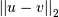# scipy.spatial.distance.euclidean¶

scipy.spatial.distance.euclidean(u, v)[source]

Computes the Euclidean distance between two 1-D arrays.

The Euclidean distance between 1-D arrays u and v, is defined asParameters: u : (N,) array_like Input array. v : (N,) array_like Input array. euclidean : double The Euclidean distance between vectors u and v.

#### Previous topic

scipy.spatial.distance.dice

#### Next topic

scipy.spatial.distance.hamming